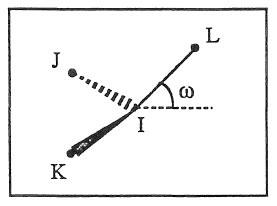$$\renewcommand{\AA}{\text{Å}}$$

# improper_style inversion/harmonic command

## Syntax

improper_style inversion/harmonic


## Examples

improper_style inversion/harmonic
improper_coeff 1 18.776340 0.000000


## Description

The inversion/harmonic improper style follows the Wilson-Decius out-of-plane angle definition and uses an harmonic potential:

$E = K \left(\omega - \omega_0\right)^2$

where $$K$$ is the force constant and $$\omega$$ is the angle evaluated for all three axis-plane combinations centered around the atom I. For the IL axis and the IJK plane $$\omega$$ looks as follows:Note that the inversion/harmonic angle term evaluation differs to the improper_umbrella due to the cyclic evaluation of all possible angles $$\omega$$.

The following coefficients must be defined for each improper type via the improper_coeff command as in the example above, or in the data file or restart files read by the read_data or read_restart commands:

• $$K$$ (energy)

• $$\omega_0$$ (degrees)

If $$\omega_0 = 0$$ the potential term has a single minimum for the planar structure. Otherwise it has two minima at +/- $$\omega_0$$, with a barrier in between.

## Restrictions

This improper style can only be used if LAMMPS was built with the MOFFF package. See the Build package doc page for more info.

none